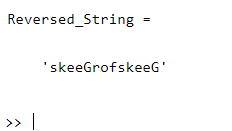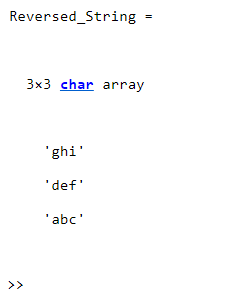GeeksforGeeks App
Open AppBrowser
Continue

# How to reverse a string in MATLAB?

In this article, we are going to discuss the “Reversing of a string” in MATLAB which can be done in multiple approaches that are illustrated below:

## Method 1: Using Built-in Functions

By using MATLAB built-in functions like reverse(), flip() and fliplr(). Built-in functions can be defined as – ” The functions which are already defined in programming framework and perform a specific task”.

## Using reverse()

The reverse() function is used to reverse the order of characters in the specified strings.

Syntax: reverse(string)

Parameters: This function accepts a single parameter.

string: This is the specified string whose characters are going to be reversed.

Example:

## Matlab

 `% MATLAB code for reverse()``% Initializing a string``string = ``'GeeksforGeeks'``;`` ` `% Calling the reverse() function``% over the above string to``% reverse its characters``Reversed_String = reverse(string)`

Output:## Using flip()

The flip() function is used to flip the order of the specified string.

Syntax: flip(A)

flip(A, dim)

Here,

flip(A) function is used to return an array of the same size as A, but with the reversed order of the elements.

flip(A, dim) function is used to reverses the order of the elements of A along dimension dim. For example, if A is a matrix, then flip(A,1) reverses the elements in each column, and flip(A,2) reverses the elements in each row.

Example 1:

## Matlab

 `% MATLAB code for reverse``% string using flip()``% Initializing a string``string = ``'GeeksforGeeks'``;`` ` `% Calling the flip() function``% over the above string to``% reverse its characters``Reversed_String = flip(string)`

Output:Example 2:

## Matlab

 `% MATLAB code for reverse``% string using flip()``% Initializing a string``string = [``'a'` `'b'` `'c'``          ``'d'` `'e'` `'f'``          ``'g'` `'h'` `'i'``];`` ` `% Calling the flip() function``% over the above string along with``% first dimensions to``% reverse its elements``Reversed_String = flip(string, 1)`

Output:## Using fliplr()

The fliplr() function is used to flip the specified string from left to right.

Syntax: fliplr(String)

Parameters: This function accepts a parameter.

String: It is the specified string whose characters are going to be flipped from left to right.

Example:

## Matlab

 `% MATLAB code for reverse ``% string using fliplr()``% Initializing a string``string = ``'GeeksforGeeks'``;`` ` `% Calling the fliplr() function``% over the above string to``% flip its characters from left ``% to right``Reversed_String = fliplr(string)`

Output:## Method 2:  Using the end keyword

In this approach, the string is reversed using the process below. Here “end” means the last element in the array, so “end-1” is the next to the last element in the array

Example:

## Matlab

 `% MATLAB code for reverse string ``% Initializing a string``string = ``'GeeksforGeeks'``;`` ` `% Reversing the above string``Reversed_String = string(``end``:-1:1)`

Output:## Method 3: Using for loop

A basic for loop in MATLAB is often used to assign to or access array elements iteratively. We can reverse a string using for loop also.

## Matlab

 `% MATLAB code for reverse ``% string using for loop``% Initializing a string``String = ``"GeeksforGeeks"``;`` ` `% using FOR loop:``NewString = [];``for` `i = 1:length(String)``    ``NewString = [NewString, String(``end``-i+1)];``end`` ` `% Getting the reversed string``Reversed_String = NewString`

Output:My Personal Notes arrow_drop_up